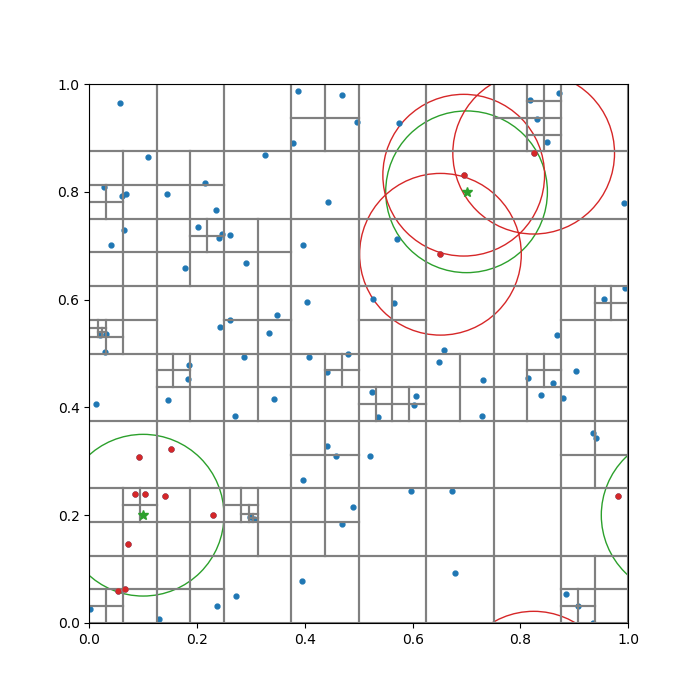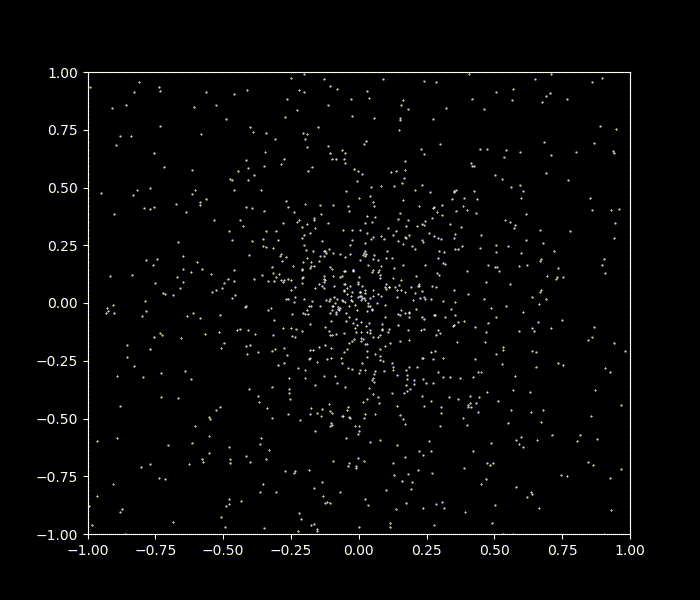## OctreeBH.jl

Barnes–Hut octree
Author huchiayu
Popularity
2 Stars
Updated Last
2 Years Ago
Started In
March 2021

# OctreeBH

`OctreeBH` is an implementation of octree for solving N-body problems using the Barnes–Hut approximation. Namely, tree nodes carry information that can be summed or compared in an efficient way. The package provides two main functionalities: (1) finding particles within a given radius (neighbor search) and (2) calculating gravitational acceleration in an N-body system. Neighbor search can be done in either the "gather" or "scatter" approach, which is particularly useful in smoothed-particle hydrodynamics. Gravitational acceleration adopts the monopole approximation, i.e., distant particles are clustered together and treated as a point mass located at their center of mass. Boundary conditions can be open, periodic, or mixed (e.g. periodic in x & y while open in z). Spatial dimension (N) can be any arbitrary positive integer.

# Quick Start

Finding particles within a searching radius `hsml0` centered at `x`:

``````using OctreeBH
using StaticArrays

N = 3 #spatial dimension
T = Float64

Npart = 100000 #number of particles
boxsizes = @SVector(ones(N)) #for periodic B.C.
topnode_length = @SVector(ones(N)) #size of the top tree node
center = topnode_length .* 0.5 #center of the top tree ndoe

hsml = ones(Npart) .* hsml0 #particle smoothing length
mass = ones(Npart) #particle mass

#randomly distributing particles
X = [@SVector rand(N) for _ in 1:Npart]

#store particle data into the struct "Data"
part = [Data{N,T}(X[i], i, hsml[i], mass[i]) for i in 1:Npart]

#build the tree
tree = buildtree(part, center, topnode_length);

x = @SVector rand(N) #search center

#get indices of the gather neighbors
idx_ngbs_g = get_gather_ngb_tree(x, hsml0, tree, boxsizes)

#get indices of the scatter neighbors
idx_ngbs_s = get_scatter_ngb_tree(x, tree, boxsizes)

#for constant hsml, the two ngbs are identical
@show length(idx_ngbs_g), length(idx_ngbs_s)
``````

# Example

The example code visualization.jl visualizes the (quad)tree structure for a given particle configuration in 2D. It also illustrates the difference between the gather neighbors (lower left) and scatter neighbors (upper right), which are identical in this particular case as the smoothing length (search radius) is constant.The example code nbody.jl solves a gravitational N-body system using the leapfrog integration scheme.# Author

Chia-Yu Hu @ Max Planck Institute for Extraterrestrial Physics (cyhu.astro@gmail.com)

### Used By Packages

No packages found.